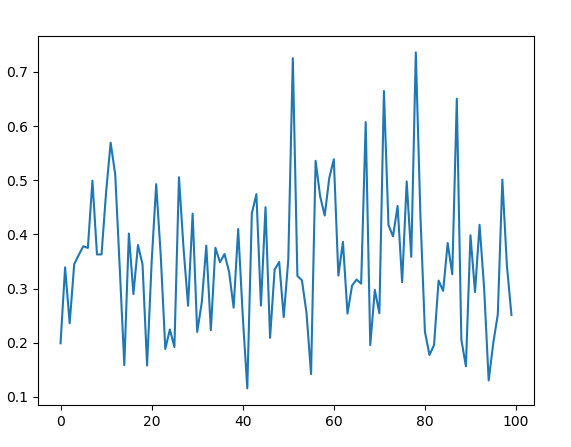# random.betavariate() method in Python

`betavariate()` is an inbuilt method of the `random` module. It is used to return a random floating point number with beta distribution. The returned value is between 0 and 1.

Syntax : random.betavariate(alpha, beta)

Parameters :
alpha : greater than 0
beta : greater than 0

Returns : a random beta distribution floating number between 0 and 1

Example 1:

 `# import the random module ` `import` `random ` ` `  `# determining the values of the parameters ` `alpha ``=` `5` `beta ``=` `10` ` `  `# using the betavariate() method ` `print``(random.betavariate(alpha, beta)) `

Output :

`0.5148685287422776`

Example 2: We can generate the number multiple times and plot a graph to observe the beta distribution.

 `# import the required libraries ` `import` `random ` `import` `matplotlib.pyplot as plt ` ` `  ` `  `# store the random numbers in a  ` `# list ` `nums ``=` `[] ` `low ``=` `10` `high ``=` `100` `mode ``=` `20` ` `  `for` `i ``in` `range``(``100``): ` `    ``temp ``=` `random.betavariate(``5``, ``10``) ` `    ``nums.append(temp) ` `     `  `# plotting a graph ` `plt.plot(nums) ` `plt.show()`

Output :My Personal Notes arrow_drop_upCheck out this Author's contributed articles.

If you like GeeksforGeeks and would like to contribute, you can also write an article using contribute.geeksforgeeks.org or mail your article to contribute@geeksforgeeks.org. See your article appearing on the GeeksforGeeks main page and help other Geeks.

Please Improve this article if you find anything incorrect by clicking on the "Improve Article" button below.

Article Tags :

1

Please write to us at contribute@geeksforgeeks.org to report any issue with the above content.